Name:    Chapter 3 Pre Test

Multiple Choice
Identify the choice that best completes the statement or answers the question.

1.

Evaluate the function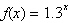at. Round to 3 decimal places.
 a. 5.287 b. 2.572 c. 4.68 d. 1.69 e. 20.055

2.

What is the value of the function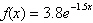at? Round to 3 decimal places.
 a. 1.398 b. 0.676 c. 1.795 d. –7.747 e. 0.848

3.

What is the value of the function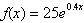at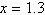? Round to 3 decimal places.
 a. 26.492 b. 37.296 c. 42.051 d. 7.597 e. 82.783

4.

Use the One-to-One Property to solve the following equation for x.a.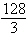b.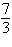c.d.e. 2

5.

Use the One-to-One Property to solve the following equation for x.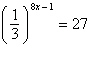a.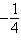b.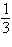c.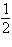d.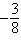e.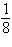6.

Rewrite the logarithmic equation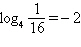in exponential form.
 a.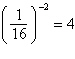b.c.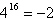d.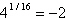e.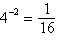7.

Evaluate the function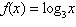at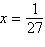without using a calculator.
 a. –2 b. –3 c.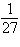d. 27 e. –4

8.

Identify the value of the function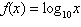at. Round to 3 decimal places.
 a. 3.118 b. 4.118 c. 2.618 d. 6.028 e. 3.618

9.

Write the exponential equation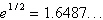in logarithmic form.
 a.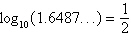b.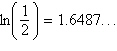c.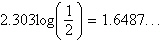d.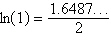e.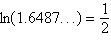10.

Identify the x-intercept of the function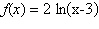.
 a.b. The function has no x-intercept. c.d.e.11.

Rewrite the logarithmin terms of the common logarithm (base 10).
 a.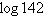b.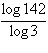c.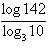d.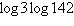e.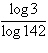12.

Rewrite the logarithmin terms of the natural logarithm.
 a.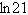b.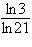c.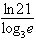d.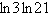e.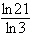13.

Evaluate the logarithm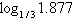using the change of base formula. Round to 3 decimal places.
 a. 0.630 b. 0.273 c. –0.692 d. –1.745 e. –0.573

14.

Find the exact value of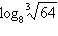without using a calculator.
 a.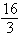b. –1 c.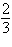d.e.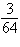15.

Simplify the expression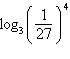.
 a. 1 b. The expression cannot be simplified. c. –108 d. –12 e. 4

16.

Put the expressions in the appropriate order: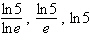.
 a.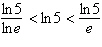b.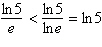c.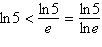d.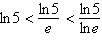e. The expressions are equivalent.

17.

Solve for x: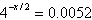. Round to 3 decimal places.
 a. 13.291 b. 10.518 c. –3.794 d. –13.291 e. 7.587

18.

Solve for x: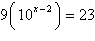. Round to 3 decimal places.
 a. 2.407 b. –1.362 c. 0.407 d. 1.362 e. no solution

19.

An initial investment of \$9000 grows at an annual interest rate of 5% compounded continuously. How long will it take to double the investment?
 a. 1 year b. 13.86 years c. 14.86 years d. 13.40 years e. 14.40 years

20.

The population P of a bacteria culture is modeled by, where t is the time in hours. If the population of the culture was 5800 after 40 hours, how long does it take for the population to double? Round to the nearest tenth of an hour.
 a. 59.3 hours b. 94.5 hours c. 9.6 hours d. 57.5 hours e. 92.7 hours